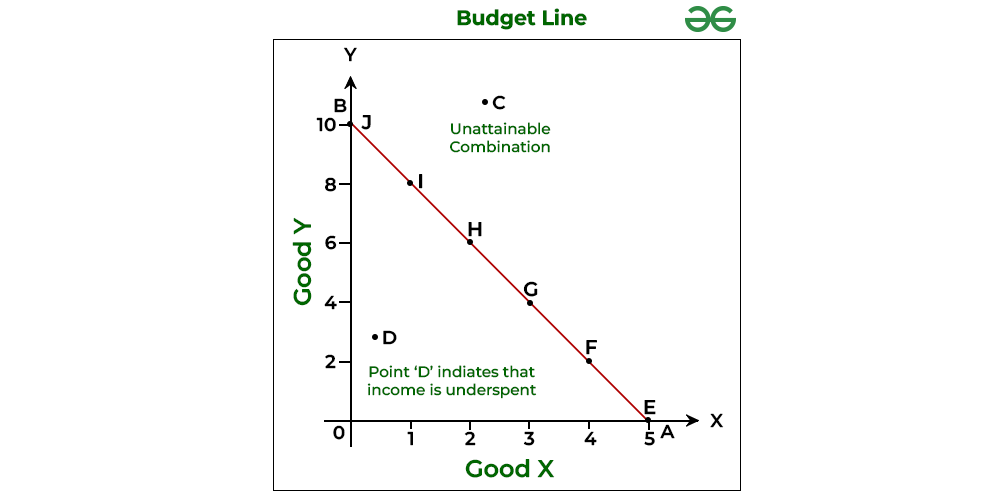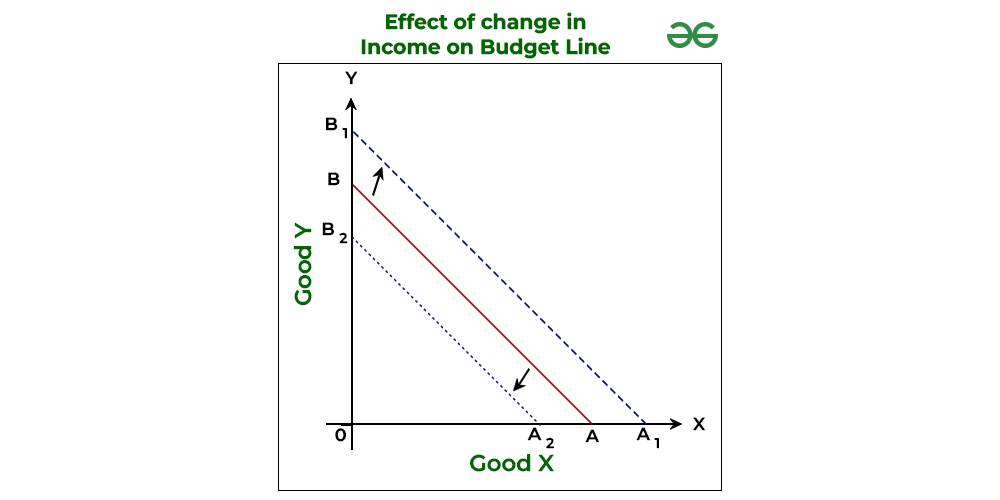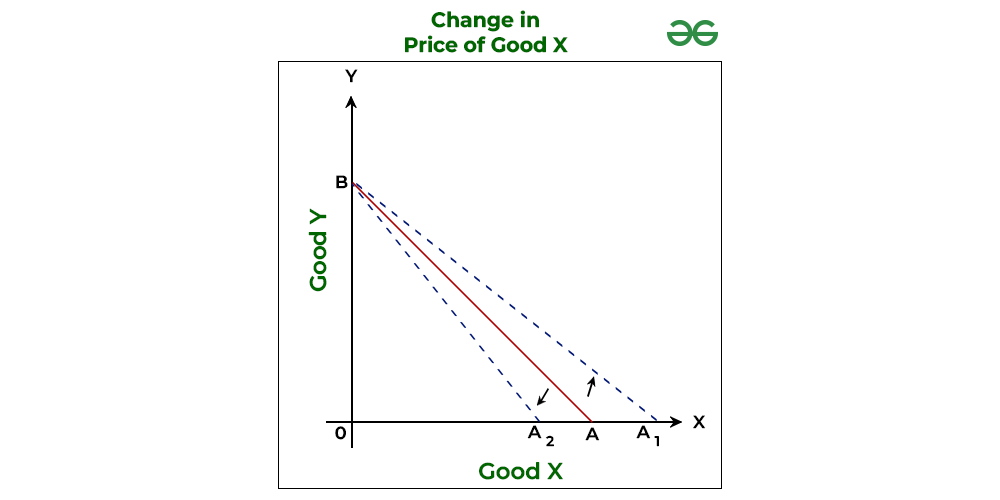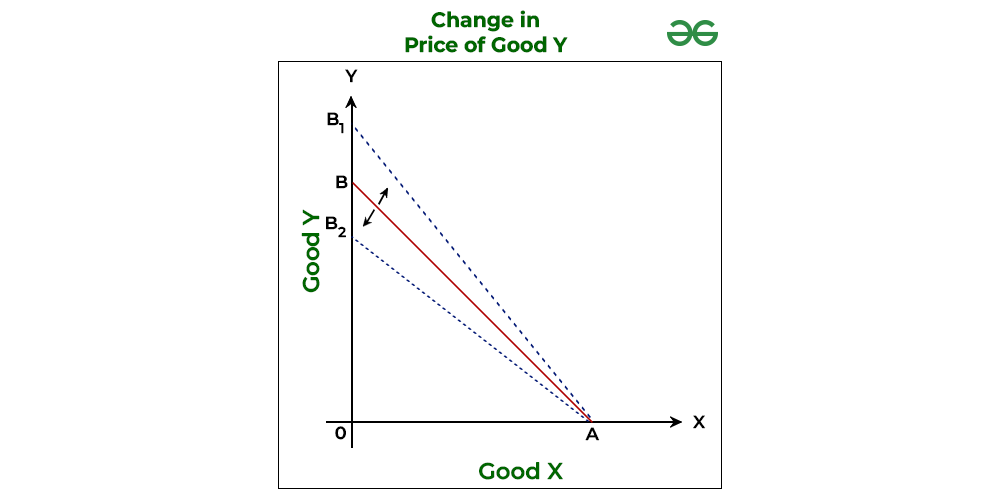GFG App
Open AppBrowser
Continue

# Budget Line: Meaning, Properties, and Example

### What is Budget Line?

The term budget line refers to a graphical representation of all the potential combinations of two commodities that can be bought within a certain income and price, and all of these combinations provide the same satisfaction level. It comes with the condition that the cost of each combination must be less than or equal to the consumer’s money income. Simply put, a budget line is the locus of various combinations of two goods a consumer consumes and whose cost is equal to his income. Other names of Budget Line are Price Line, Price Opportunity Line, Budget Constraint Line, or Price Income Line.

For example, a consumer’s income is ₹10 and he wants to spend the money on two commodities, say X and Y and both of these goods are priced at ₹5 each. Now the consumer has three options for spending his income. The first option is to buy two units of commodity X. Second option is to buy two units of commodity Y. or the third option is to buy one unit of commodity X and one unit of commodity Y. This means that the possible bundles, in this case, can be (2, 0); (0, 2); and (1, 1). Now, when all of these three bundles are represented on a graph, a downward-sloping straight line is formed which is known as a budget line.

#### Budget Set

The set of all possible combinations of the two commodities a consumer can afford to buy with his given income and price in the market is known as a budget set. In the above example, besides the three options; viz., (2, 0); (0, 2); and (1, 1), there are some other options which are available for the customer within his total income of ₹10, even if he does not spend his entire income. A budget set in this case will include all the bundles which have a total income of ₹10. The possible Consumer’s Bundles are (0, 0); (0, 1); (0, 2); (1, 0); (2, 0); and (1, 1). Hence, a consumer’s bundle is a quantitative combination of two goods which a consumer can purchase from his given income.

### Diagrammatic Explanation of Budget Line

Assume that a consumer has a total budget of ₹40, with which he can buy various combinations of Good X and Good Y. The cost of one unit of Good X is ₹8 and the cost of one unit of Good Y is ₹4 respectively. Now, the potential set of combinations that can be purchased by the consumer are:In the above graph, Good X is represented on the X-axis and Good Y on the Y-axis. At one extreme of the graph; i.e., Point E, the consumer can purchase 5 units of Good X by spending the entire income; i.e., ₹40 on it. The other extreme point of the graph; i.e., Point J, the consumer can purchase 10 units of Good Y by spending the entire income on Good Y. Between these two extreme points, there are other combinations; i.e., Point F, G, H, and I. By joining the points from E to J, a straight line ‘AB’ is formed, which is known as the Budget Line or Price Line.

Therefore, in the above graph, AB is the Budget Line and OAB is the Budget Set. Also, every point on the Budget Line indicates different bundles of Good X and Good Y that the consumer can purchase by spending his entire income of ₹40 at the given prices of goods.

#### Important Observations:

1. The slope of Budget Line AB is downwards. It is because more of one good can be bought by decreasing some units of the other good.
2. The bundles whose cost is exactly equal to the consumer’s money income, such as combinations E to J, lie on the budget line.
3. The bundles whose cost is less than the consumer’s money income, such as combination D, show underspending by the consumer. These bundles lie inside the budget line.
4. The bundles whose cost is more than the consumer’s money income, such as combination C, show unattainable combinations; i.e., the combinations which are not available to the consumer. These bundles lie outside the budget line.
5. Budget Line is more relevant than Budget Set when one needs to determine the Consumer’s Equilibrium as it is based on the assumption that the consumer spends his entire income.

### Algebraic Expression of Budget Line

The Budget Line can be expressed as an equation:

M = (PX x  QX) + (PY  x  QY)

Where,

M = Individual’s Income

PX = Price of Commodity X

Qx = Quantity of Commodity X

PY = Price of Commodity Y

QY = Quantity of Commodity Y

All points on the Budget Line AB show those bundles whose cost is exactly equal to M.

### Slope of Budget Line

A curve’s slope is usually determined by dividing the change in a variable on the vertical axis (Y-axis) by the change in a variable on the horizontal axis (X-axis). In the previous example of Good X and Good Y, the slope of the budget line will be the number of units of Good Y, that a consumer is willing to sacrifice for one extra unit of Good X.As seen in the above graph, as we move downwards the slope, 2 units of Good Y need to be sacrificed to gain 1 unit of Good X. Therefore, the Slope of Budget Line is.

* The numerator of a budget line will always be negative as it shows the number of units to be sacrificed. However, to have better analysis, absolute value is considered.

The Slope of a Budget Line is denoted by the Market Rate of Exchange or MRE. Market Rate of Exchange is the rate at which one good is sacrificed in the market in order to obtain one additional unit of other good. Also, the slope of a budget line or MRE is equal to the Price Ratio of two goods.

#### Price Ratio

The price of the good on X-axis divided by the price of the good on Y-axis is known as Price ratio. For example, if Good X is shown on horizontal axis and Good Y on vertical axis, then#### Why Slope of the Budget Line is represented by Price Ratio?

A point on the budget line represents a bundle, which a consumer can purchase by spending his entire income. Therefore, if a consumer wants to consume one more unit of a good (say, Good Y), he has to sacrifice some amount of other good (say, Good X). In the above example, Good X is priced at ₹8 (Px) and Good Y at ₹4 (PY). So, if the consumer wants to gain 1 unit of Good X, he will have to reduce his expenditure on Good Y by ₹4. Therefore, the consumer will have to sacrifice 2 units of Good Y to gain 1 unit of Good X, which means that the consumer will have to give \frac{P_X}{P_Y} units of Good Y to gain one unit of Good X. Here,is the price ratio between Good X and Good Y.

Hence, it can be said that Price Ratio indicates the Slope of the Budget Line.

### Properties of Budget Line

The two major properties of a Budget Line are as follows:

1. Budget Line slopes Downward: The slope of a budget line is negative. It means that as more of one good is bought by reducing some units of the other good, the slope of the budget line goes downwards.
2. Budget Line is a Straight Line: We know that the slope of the Budget Line is represented by the Price Ratio, which is constant throughout; therefore, the Budget Line is a straight line.

### Shift in Budget Line

While drawing a budget line, it is assumed that the income of the consumer and the price of the commodities is constant. Therefore, the need to draw a new budget line will arise when there is either a Change in the Income of the Consumer or a Change in the Price of the Commodity.

#### 1. Effect of a Change in the Income of Consumer:

While assuming that the price of Good X and Good Y in the above example remains constant, if there is a change in the income of the consumer, then the budget line will shift. When there is an increase in the income of the consumer, then he will be able to purchase more bundles of Good X and Good Y, which were not possible for him earlier. This will shift the budget line to the right from AB to A1B1. This new budget line will be parallel to the original budget line. Similarly, when there is a reduction in the income of the consumer, then the budget line will shift to the left from AB to A2B2#### 2. Effect of Change in Prices of the Commodities:

There are three cases in which the price can change. These are as follows:

i) Change in Prices of both Commodities: When the price of both goods changes, then the budget line will shift. If the price of both goods falls, then the Budget Line will shift right from AB to A1B1. However, if the price of both goods increases, then the Budget Line will shift to the left from AB to A2B2ii) Change in the Price of Commodity on the X-axis (Good X): When the price of Good X falls, then the budget line will rotate to the right from AB to A1B. It means that the new budget line will meet the Y-axis at the same point; i.e., B because the price of Good Y has not changed; however, it will touch the X-axis at point A1 because the consumer can now buy more units of Good X with his same income level. Similarly, if the price of Good X rises, then the budget line will rotate to the left from AB to A2B.iii) Change in the Price of Commodity on the Y-axis (Good Y): When the price of Good Y falls, then the budget line will rotate to the right from AB to AB1. It means that the new budget line will meet the X-axis at the same point; i.e., A because the price of Good X has not changed; however, it will touch the Y-axis at point B1 because the consumer can now buy more units of Good Y with his same income level. Similarly, if the price of Good Y rises, then the budget line will rotate to the left from AB to AB2.My Personal Notes arrow_drop_up